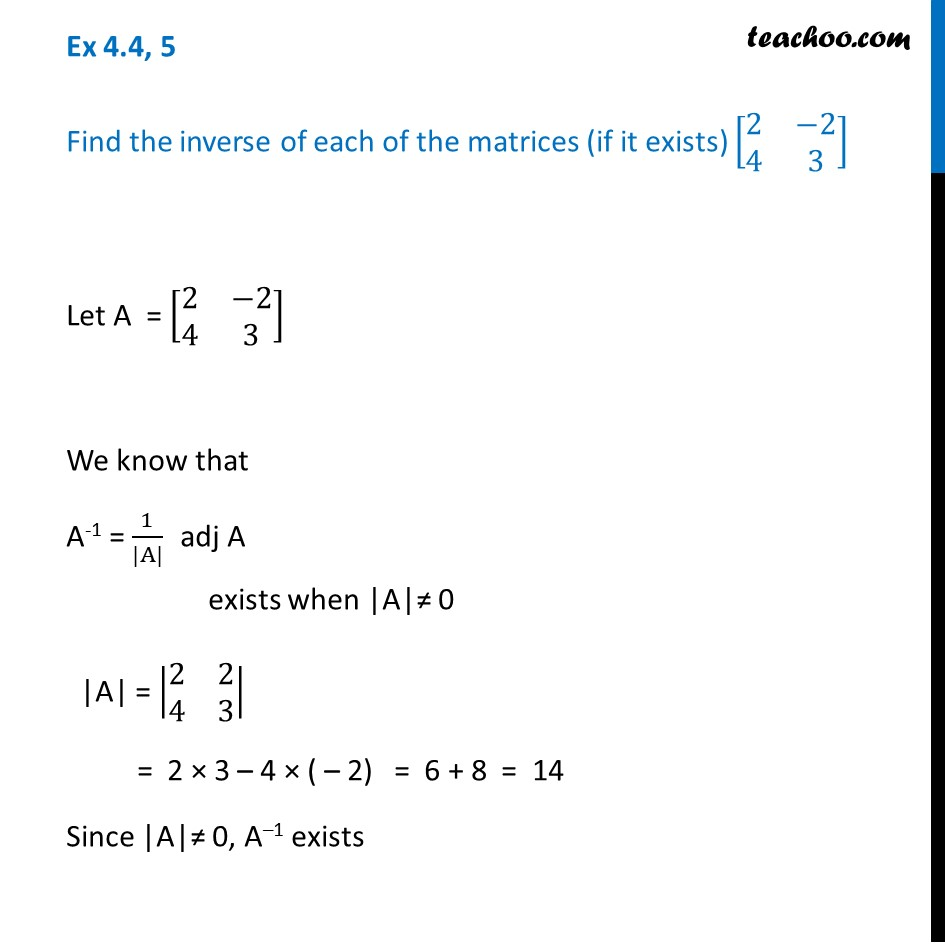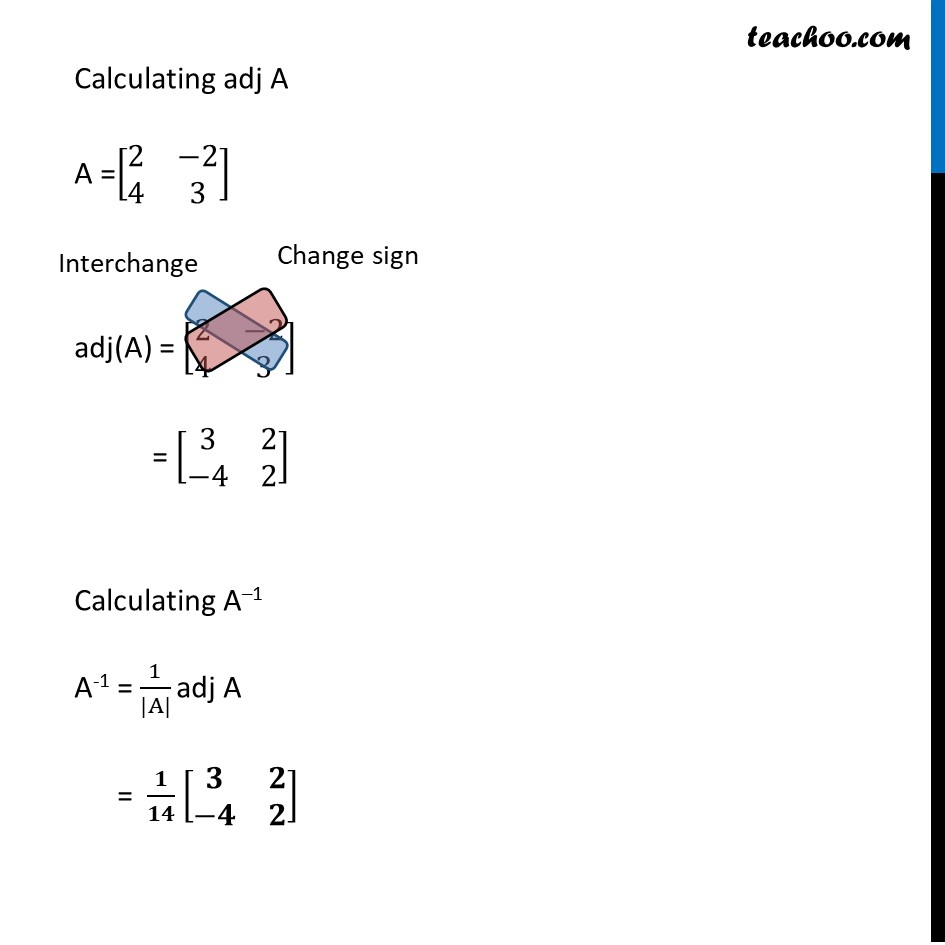Ex 4.4

Chapter 4 Class 12 Determinants
Serial order wiseLearn in your speed, with individual attention - Teachoo Maths 1-on-1 Class

### Transcript

Ex 4.4, 5 Find the inverse of each of the matrices (if it exists) [■8(2&−2@4&3)] Let A = [■8(2&−2@4&3)] We know that A-1 = 1/(|A|) adj A exists when |A|≠ 0 |A| = |■8(2&2@4&3)| = 2 × 3 – 4 × ( – 2) = 6 + 8 = 14 Since |A|≠ 0, A–1 exists Calculating adj A A =[■8(2&−2@4&3)] adj(A) = [■8(2&−2@4&3)] = [■8(3&2@−4&2)] Calculating A–1 A-1 = 1/(|A|) adj A = 𝟏/𝟏𝟒 [■8(𝟑&𝟐@−𝟒&𝟐)]